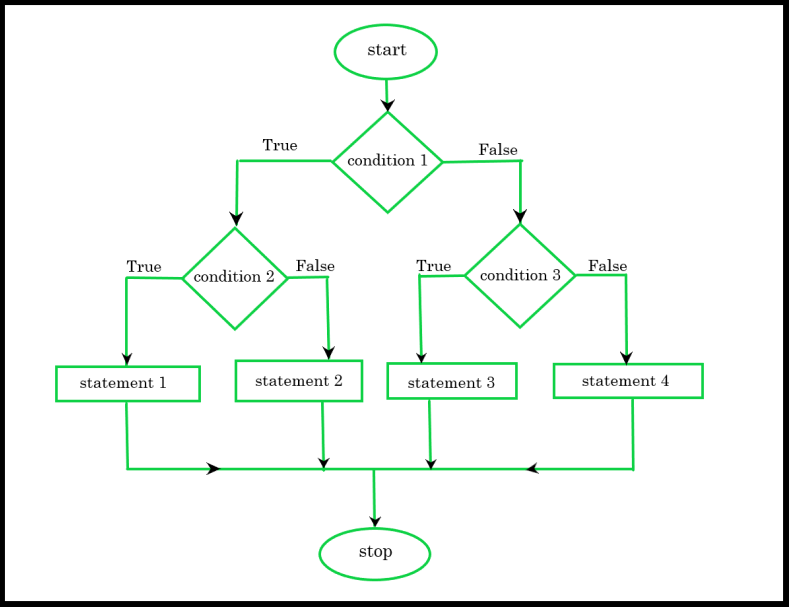# Swift – Nested if-else Statement

• Last Updated : 28 Feb, 2022

In Swift, a situation comes when we have to check an if condition inside an if-else statement then a term comes named nested if-else statement. It means an if-else statement inside another if statement. Or in simple words first, there is an outer if statement, and inside it another if – else statement is present and such type of statement is known as nested if statement. We can use one if or else if statement inside another if or else if statements.

Syntax:

```// Outer if condition
if (condition 1)
{

// inner if statement
if (condition 2)
{
// Statement 1
}
// inner else statement
else
{
// Statement 2
}
}

// Outer else statement
else
{

// inner if statement
if (condition 3)
{
// Statement 3
}

// inner else statement
else
{
// Statement 4
}
}```

Here, if the outer condition1 is true, then it will check for inner condition 2. If inner condition is true then it will proceed to statement 1 otherwise statement 2 will proceed. Similarly in outer else part will execute.

Notes: We can add else and else if statements to the inner if statement whenever required. Also, we can nest multiple layers of if-else statements inside a if statement.

Flowchart:Example 1:

## Swift

 `// Swift program illustrate the use``// of Nested if-else statement` `import` `Swift``var` `a = 100``var` `b = 200``var` `c` `= 300` `// outer if statement``if` `(a > b) {` `  ``// inner if statement``  ``if` `(a > ``c``) {``    ` `      ``// Print statement 1``      ``print``(``"100 is Greater"``)``  ``}` `  ``// inner else statement``  ``else` `{``      ``// Print statement 2``      ``print``(``"300 is Greater"``);``  ``}``}` `// outer else statement``else` `{``  ` `  ``// inner if statement``  ``if` `(b > ``c``) {``    ` `      ``// Print statement 3``      ``print``(``"200 is Greater"``)``  ``}` `  ``// inner else statement``  ``else` `{``    ` `      ``// Print statement 4``      ``print``(``"300 is Greater"``);``  ``}``}`

Output:

`300 is Greater `

Explanation : In above example, First it will check for outer if condition. If outer if condition is true then it will check for inner if statement. If outer if statement is false it then will move to outer else part and so on. Here outer if condition is false. Next it will move to outer else part and check for inner if statement i.e. if b is greater than c ( b > c ). Here it evaluates false. Hence it will move to inner else part and prints statement 4.

Example 2 :

## Swift

 `// Swift program illustrate the use of``// Nested if-else-if statement``import` `Swift`  `var` `number = 5` `// outer if statement``if` `(number >= 0) {` `  ``// inner if statement``  ``if` `(number == 0) {``    ` `      ``// Print statement 1``      ``print``(``"Number is 0"``)``  ``}` `  ``// inner else statement``  ``else` `{``    ` `      ``// Print statement 2``      ``print``(``"Number is greater than 0"``);``  ``}``}` `// outer else statement``else` `{``  ` `    ``// Print statement 3``    ``print``(``"Number is smaller than 0"``);``}`

Output :

`Number is greater than 0`

Explanation : Here we have declared a variable number with value 15. Then we have used if-else construct. If the outer if condition is true then and only then it will execute the inner if condition. In this example, the outer if condition is true hence the inner if block is processed. In the inner if condition, we again have a condition that checks if number is equals to 0 or not. When inner condition is true, then it will process the print statement 1  and If inner if statement is false then it will process the print statement 2. In this case, the inner if condition is false  hence it will move to else part and prints statement 2 and the value is printed on the output screen.

My Personal Notes arrow_drop_up